## Example Questions

### Example Question #7 : Negative Numbers

Find the value of.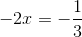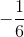Explanation:

To solve for, divide each side of the equation by -2.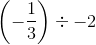is the same as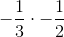which is POSITIVE### Example Question #1 : How To Divide Negative Numbers

What is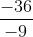?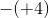45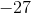Explanation:

A negative number divided by a negative number always results in a positive number.divided byequals. Since the answer is positive, the answer cannot beor any other negative number.

### Example Question #2 : How To Divide Negative Numbers

Solve for: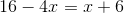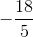Explanation:

Subtractfrom both sides: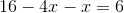, or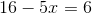Next, subtractfrom both sides: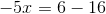, or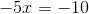Then, divide both sides by: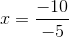Recall that division of a negative by a negative gives you a positive, therefore: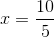or### Example Question #71 : Integers

Solve for: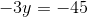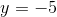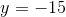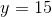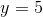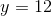Explanation:

To solve this equation, you need to isolate the variable on one side. We can accomplish this by dividing byon both sides: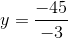Anytime you divide, if the signs are the same (i.e. two positive, or two negative), you'll get a positive result.  If the signs are opposites (i.e. one positive, one negative) then you get a negative.

Both of the numbers here are negative, so we will have a positive result:### Example Question #72 : Integers

Solve for: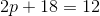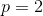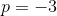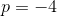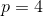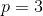Explanation:

To solve, you need to isolate the variable. We first subtractthen divide by: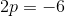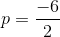When dividing, if the signs of the numbers are the same (i.e. both positive, or both negative), you yield a positive result.  If the signs of the numbers are opposites (i.e. one of each), then you yield a negative result.

Therefore:Tired of practice problems?

Try live online GRE prep today.